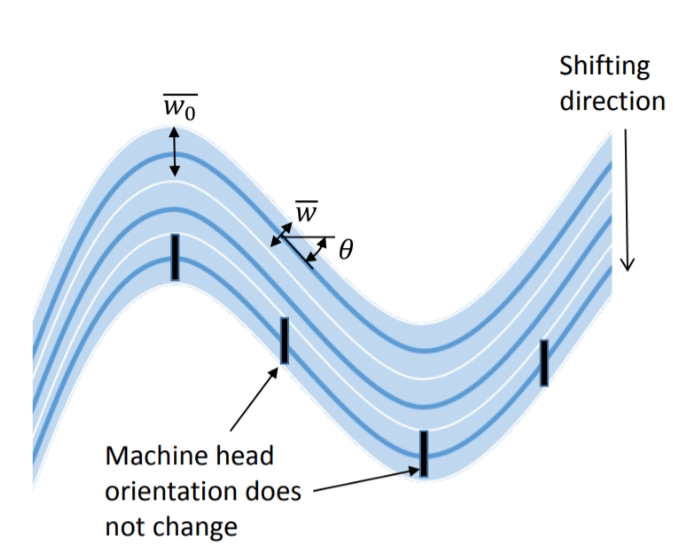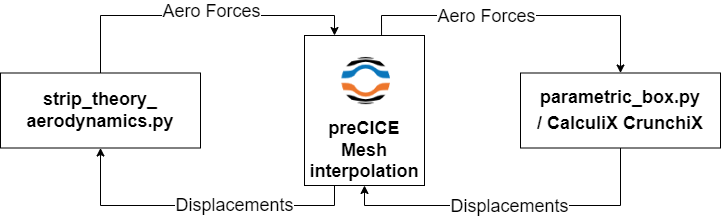Dapta

# Automated FEM analysis and output processing with Python and CalculiX CrunchiX (ccx)

This is a follow-on from my last post, where I covered parametric model creation using Python and Calculix GraphiX. Here, we will automate adding composite material properties to our model, running a static analysis in CalculiX CrunchiX (CCX) and reading & plotting the outputs — so that we can actually run a parametric study really fast. Read-on to find out how…

Note: The following will all sound very theoretical unless you try it out yourself — there is a copy of the example source code on github.com (make sure you use v0.0.2 for this post, as v0.0.1 is a snapshot of the previous post example code).

You can also get a quick video run-through of the code updates on Youtube, where I explain the inputs/outputs and demo the parametric study execution.

#### Example part 2: Automated FEM analysis and output processing

Here are the steps to go from our last example to running a parametric composite design study on our simple wing FEM:

##### 1. Define the process inputs and outputs

We keep all our design inputs from last time and add additional composite material design parameters (ply types, ply reference orientations and layup sequences). In addition, we now want to be able to generate a Calculix CrunchiX input deck, which of course has a different format from the Nastran deck we used last time. So we need a Calculix Analysis master file that includes all the model data that will not be parameterized (e.g. the actual ply material stiffness properties and loading sequence do not change in this example).

In terms of outputs, we now want to recover certain quantities of interest from the analysis output and make them available for further processing, for example for plotting in Python. In this example, we will apply a static shear force to the tip of our cantilever box model. The quantities that we want to recover are the average tip displacements and rotations — we will need to calculate the average ourselves from the raw FEM output data.

The output from our parametric study will be the changes in these tip deflections as a function of our parameter value, which in this case will be the main ply fibre direction. To generate this output, we need to run the static analysis multiple times and save the outputs after each run (or iteration).

##### 2. Define the steps required to get from our inputs to the outputs

Again, we start at the end of the process and work backwards:

• To generate the parametric study data, we need to save the input parameter values and recover the processed outputs from multiple analyses.
• To generate the processed outputs from an analysis, we need to be able to read and process the data from an analysis output file.
• To generate the analysis output file, we need to execute the FEM solver on the input deck that is output from the parametric FEM model script.

We can now extend the automation process plot from our last blog post to look like this:

##### 3. Implement the steps in a Python script

There are of course many ways to implement these updates — here is my take on it.

As last time, the full code can be found on Github.

##### 4. Execute the Python script and verify the outputs

The video now shows the actual full process execution and parametric study output. Overall, the tip deflection trends make sense and the deflection magnitude are also sensible:

In practice, we should of course add mode validations and tests to this script to make sure the results are as expected. For example, from an engineering analysis perspective, we may want to know if the FEM mesh refinement that we used is sufficient — and in fact we can do this quite easily now if we set our parametric variable to the mesh refinement input variables instead of the composite orientation. I implemented this as an additional study example in the code (Note: there seems to be a bug in CalculiX GraphiX if the mesh density is increased to 100 nodes or more on any one geometry line — so try to keep below that!).

#### Finally…

We now have the full automated process in place, including: model geometry generation, meshing, analysis, post processing and automated repetition of this process with different input values.

In the process, our Python script has grown quite a bit and could probably do with a bit of cleaning-up — especially if I wanted to continue using and expanding it with more options in the future (e.g. more parameters, more solvers etc.). We may also want to (finally!) wrap an optimiser around this … more on that next time!

Keywords

## Related PostsTutorial### Dynamic aeroelastic flutter and divergence analysis with Python and Calculix

We add a dynamic aeroelastic stability analysis to our parametric wing analysis, so that we can calculate critical flutter and divergence flight velocities. We extract mass and stiffness information from our CalculiX model and then solve a complex eigenvalue problem with the help of the Scipy sparse matrix library.Technology### Design and analysis of RTS steered-fibre composites

In this post, we take a brief look at steered-fibre composites and particularly RTS composites. What are they? Why do we want to use them? And how do we design with them?